#Quality Digest

Featured Product
This Week in Quality Digest Live
Operations Features
Del Williams
Options to address the risk of combustible dust explosions for NFPA 61 compliance
Gleb Tsipursky
Tess Malone
So what does?
Mark Hembree
Is collaboration overrated?
Matt Fieldman
German system offers tips for U.S. counterparts
Operations News
Safe trading practices to secure supply chain activities
VSL hosts special edition of show at new center in Rotterdam
Latest line touts comprehensive coverage, ease of use
Same price, double the resolution and accuracy
Former service partner provides honing and deep-hole drilling solutions
Low-cost prevention of catastrophic failures
Diagnoses LVDT failures, disconnects, or short circuits
Quality doesn’t have to sacrifice efficiency
January 24–26, 2023, Booth 1701, San Diego Convention CenterBio

Operations

## Confidence Intervals for Proportions and Poisson Means

### Attribute data are better than no data, but that’s about the best you can say of them

Published: Monday, April 4, 2016 - 23:00

Experiments that might require a handful of real-number measurements (variables data) could need hundreds or more attribute data for comparable power, i.e., the ability to determine whether an experiment improves performance over that of a control. Sample sizes needed for ANSI/ASQ Z1.4 (for inspection by attributes) are similarly much larger than sample sizes for ANSI/ASQ Z1.9 (for inspection by variables).

One application of attribute data is the estimation of the nonconforming fraction (p) from a process. The binomial distribution is the standard model in which p is the probability that each of n items will or will not have a certain attribute (such as meeting or not meeting specifications). The probability p is assumed to be identical for every item in the population; that is, every item has the same chance of being nonconforming. In addition, the sample n is assumed to come from an infinite population. That is, removal and inspection of an item does not change the probability that the next one will have the attribute in question.

If the latter assumption is not met, the hypergeometric distribution must be used. A process is assumed to be an infinite population (hence the use of the binomial distribution for the np and p control charts), but a specific lot is not. If the lot is much larger than the sample, though, the binomial distribution is a good enough approximation to the hypergeometric.

Given a sample of n items, x of which are nonconforming, find a 100(1–α)-percent confidence interval [pL, pU] for the nonconforming fraction p. This is achieved by finding-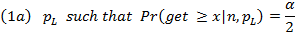where Pr is the cumulative binomial probability. The equations can be rewritten to use the cumulative binomial probability in its customary form, which reflects the chance of getting fewer than or equal to a certain number of nonconformances.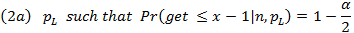As an example, the 95-percent confidence interval for the nonconforming fraction is [0.017,0.074] for eight nonconforming pieces in a sample of 208.1 The cumulative binomial probability of getting 7 or fewer nonconformances given pL = 0.017 is 0.973, which is close to the target of 0.975. Adjustment of pL to get the third or fourth significant figure could doubtlessly center the result on 0.975. The cumulative probability of getting 8 or fewer nonconformances given pU = 0.074 is similarly 0.0261.

We are, as a practical matter, interested primarily in the upper confidence limit because we don’t care how few nonconformances we get. In this case, we can assure our internal or external customer with 97.5-percent confidence that the quality is no worse than 7.4-percent nonconforming.

It is far more convenient and accurate, however, to use the relationship between the binomial, t, and F distributions to find the confidence limits for the nonconforming fraction. The equations are as follows, where the first two arguments for the F statistic are the numerator and denominator degrees of freedom, and the third is its quantile. Statistical software such as Minitab or StatGraphics, and also the F.INV function in Excel, can return F with as many significant figures as desired, so there is no need to iterate for a solution with the first equation set.In the example, F402;16;0.975 = 2.3366 and then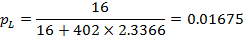F400;18;0.025 = 0.56004 and then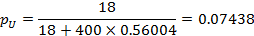The Poisson distribution is meanwhile the standard model for defects among a given number of items, or a given area (e.g., defects per square meter). It assumes, like the binomial distribution, that the mean or expected defect count (λ) is uniform throughout the population, and that defects are independent of one another. The arrivals (e.g., defects or other attributes of interest, such as the number of cars to drive through an intersection at a specific time of day) are emphatically random arrivals.

It is similarly easy to get confidence limits for the Poisson mean λ because of the relationship between it, the gamma distribution, and the chi square distribution. The chi square distribution is, in fact, a special case of the gamma distribution. In this case,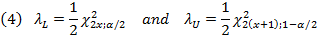As an example, if x = 6 defects, the 95-percent confidence interval for the Poisson mean is [2.2,13.1] per reference.2 The 0.025 quantile of the chi square distribution with 12 degrees of freedom is 4.404, and the 0.975 quantile with 14 degrees of freedom is 26.120. ThenWe can confirm this as follows: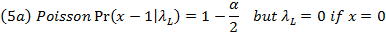In this case, the cumulative Poisson probabilities of getting 5 pieces given λ=2.202 and 6 defects given λ=13.06 are 0.9750 and 0.0250 respectively.

This article should have hopefully provided the reader with a simple way to find confidence intervals for the means of the binomial and Poisson distributions, but also note the width of the intervals in the examples. This underscores the takeaway that, although attribute data are better than no data, they lack the information that comes with numerical measurements.

References
1. Beyer, William H. CRC Standard Probability and Statistics Tables and Formulae. (CRC Press, 1991), Section III.3, “Confidence Limits for Proportions.”
2. Beyer, William H. CRC Standard Probability and Statistics Tables and Formulae. (CRC Press, 1991), Section III.4, “Confidence Limits for the Expected Value of a Poisson Distribution.”

### Discuss